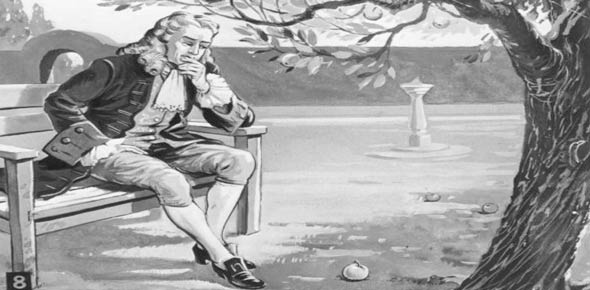# Mrs. Lawrence's Laws Of Motion Quiz

5 Questions | Attempts: 130
ShareSettingsNewton's Laws, inertia, weight, etcThis is your description.

• 1.
A push or a pull is called a ______________ and is measured in _________________.
• A.

Weight; kilograms

• B.

Force; kilograms

• C.

Weight; pounds

• D.

Force; newtons

• 2.
If a force of 50N acts on a 5 kg object, its acceleration is equal to ______ meters per second squared.
• A.

55

• B.

45

• C.

250

• D.

10

• 3.
A body at rest tends to stay at rest is called _______________,
• A.

Equilibrium

• B.

Newton's first law

• C.

Newton's second law

• D.

Newton's third law

• 4.
To find the horizontal force acting on an object that is being pulled by a rope at an angle of 50 degrees with the ground, you would use ______________.
• A.

The Pythagorean theorem

• B.

Sine

• C.

Cosine

• D.

Tangent

• 5.
The resistance an object has to a change in its motion is called ______________,
• A.

Equilbrium

• B.

Inertia

• C.

Momentum

• D.

Drag

## Related TopicsBack to top
×

Wait!
Here's an interesting quiz for you.